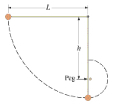# A small rubber ball is attached to a horizontal string of length L whose other end is fixed, as...

## Question:

A small rubber ball is attached to a horizontal string of length L whose other end is fixed, as shown.

a. The ball is released. Calculate its speed at the lowest point of its path? (Use the following as necessary: G and L.)

b. A peg is positioned a distance H directly below the point of attachment of the string. If H=0.82L, Calculate the speed of the ball when it reaches the top of its circular path about the peg? (Use the following as necessary: G and L.)

How would you solve for V?## Energy Conservation Principle:

The energy conservation principle consists of a physical law that states that for a closed system (no external forces work over the system), the total energy of the system will be conserved. Conservation of energy states that the energy is not created nor destroyed, it only transforms. This means that inside a system, there exists energy transformations, but the overall total energy of the system will remain the same. The total energy of a system can be determined by the sum of the total kinetic and potential energy of the system.

$$E_t=K_e+U_e\\ E_t=0.5mv^2+mgh$$

#### Part a):

In order to determine the speed at the lowest point of the pendulum, we can use the energy conservation principle, as no external forces seem to act over the system. Initially, the ball will have potential energy stored within, and as it descends, it will completely convert to kinetic energy. We solve for velocity.

Variable identification:

• Mass of the bob: {eq}m {/eq}
• Acceleration due to gravity: {eq}g {/eq}
• Length of the pendulum: {eq}L {/eq}
• velocity of the bob: {eq}v {/eq}
• Initial Energy: {eq}E_i {/eq}
• Final Energy: {eq}E_f {/eq}
• Potential Energy: {eq}U_e {/eq}
• Kinetic Energy: {eq}K_e {/eq}

\begin{align} E_i &= E_f\\ U_e &= K_e\\ mgL &= \frac{1}{2}mv^2\\ 2gL &= v^2\\ v &= \sqrt{2gL} \end{align}

Therefore, we determined that the velocity of the bob at the end of the string will depend only on the length of the pendulum, as the acceleration due to gravity will be constant.

#### Part b):

The ball will follow a perfectly circular path, as such, we can define the ball moving in a uniform circular motion. This time we have new variables to work with:

New Variables:

• Distance from the pivot to the peg: {eq}H {/eq}
• Radius of the formed circle: {eq}R {/eq}
• Centripetal force: {eq}F_c {/eq}
• Acceleration: {eq}a_c {/eq}
• Minimum velocity: {eq}V_H {/eq}

\begin{align} R &= L-H\\ R &= L-0.82L\\ R &= 0.18L\\ \end{align}

Using Newton's 2nd law applied to uniform circular motion, we can define that the minimum velocity necessary for the ball to complete the loop at the top of the peg will be defined by the following equation:

\begin{align} F_c &= ma_c\\ mg &= m\dfrac{v_H^2}{0.18L} \end{align}

We define the weight as the centripetal force because the moment the string is not tensed, the only force working in the direction to the center of the circumference will be the weight of the ball. With this, we find another equation for the velocity.

\begin{align} g &= \dfrac{v_H^2}{0.18L}\\ v_H &= \sqrt{0.18gL} \end{align}

Therefore, the velocity of the bob at the top of the peg will depend on the length of the string that is still free. The position of the peg will be very important in determining the speed of the ball at that instant.